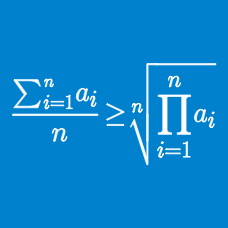Algebra

# Classical Inequality Statements: Level 2 Challenges

$\dfrac{a}{b} + \dfrac{b}{c} + \dfrac{c}{d} + \dfrac{d}{a}$

If $a, b, c$ and $d$ are any four positive real numbers, then find the minimum value of the expression above.

Let $x$ be a positive real number. Find the minimum value of $8x^{5}+10x^{-4}.$

Positive reals $a,b,c$ satisfy $abc=1$. Find the minimum value of

$\dfrac{1+ab}{1+a}+\dfrac{1+bc}{1+b}+\dfrac{1+ca}{1+c}.$

For $a,b,c>0$ and $a+b+c=6$. Find the minimum value of

$\large \left (a+\frac{1}{b} \right )^{2}+ \left (b+\frac{1}{c} \right )^{2}+\left (c+\frac{1}{a} \right )^{2}$

Find the minimum value of $\sqrt{a^2+b^2}$ when $3a+4b=15$.

×